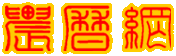共有5958人关注过本帖树形打印

# 主题：[求助]Vb6求儒略日算法程序和一个问题myyh
1楼 个性首页 | 信息 | 搜索 | 邮箱 | 主页 | UC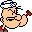加好友发短信[求助]Vb6求儒略日算法程序和一个问题Post By：2012/7/3 19:08:00

 我门外汉，刚注册进来沉这学习许剑伟老师的算法。。。。先请教一个问题，许剑伟老师 第24章 太阳位置计算中计算1992-10-13,0点,即力学时TD=JDE 2448908.5时刻的太阳位置lo = 280.46645 + 36000.76983 * t + 0.0003032 * t ^ 2我计算的结果前面的和许剑伟老师公布的一样，但后面是怎么转成201.80719的不明白，请各位老师指点下。。谢谢了。。。Lo= -2318°.19281 = 201°.80719根据许剑伟老师的算法，我用VB6编了个求儒略日的函数，可对公历任一日期计算。测试没问题，还请各位老师多指教，下面是代码：Function jd(sdate As Date) As Double  '儒略日计算    Dim y As Integer, m As Integer, d As Double    Dim a As Double, b As Double, jd As Double    y = Year(sdate): m = Month(sdate): d = Day(sdate)    If m <= 2 Then        y = y - 1: m = m + 12    End If    a = Int(y / 100)    b = 2 - a + Int(a / 4)    If y <= 1582 Then b = 0    'And m <= 10 And d <= 15 Then b = 0'儒略日格利高历界限15821015日    jd = Int(365.25 * (y + 4716)) + Int(30.6001 * (m + 1)) + d + b - 1524.5End Function函数说明：其实sdate 的数据类型应该设成Double而不是用日期型，因为儒略日是带小数的计算，这里图了方便。。。格利高历的界限还没准确到1582年的10月15日，只是作了按年的判断。。。[此贴子已经被作者于2012-7-3 23:36:26编辑过]myyh
2楼 个性首页 | 信息 | 搜索 | 邮箱 | 主页 | UC加好友发短信Post By：2012/7/3 23:41:00

 修改后的函数，格利高界限调整为15821015的12点，因需计算公园前年份，日期采用分段输入Function jd(y As Integer, m As Integer, d As Double) As Double  '儒略日计算'传统上儒略日的计数从格林尼治平午，即世界时12点开始glg = y & Format(m, "00") & Format(Int(d), "00") & d - Int(d)Dim a As Double, b As DoubleIf m <= 2 Theny = y - 1: m = m + 12End Ifa = Int(y / 100)b = 2 - a + Int(a / 4)If glg <= 15821015.5 Then b = 0 '儒略日格利高历界限15821015 12:00:00pm日jd = Int(365.25 * (y + 4716)) + Int(30.6001 * (m + 1)) + d + b - 1524.5End Functionmyyh
3楼 个性首页 | 信息 | 搜索 | 邮箱 | 主页 | UC加好友发短信Post By：2012/7/3 23:43:00

 请各位老大帮忙解答下我的问题，谢谢了。。。zhanglei1953
4楼 个性首页 | 信息 | 搜索 | 邮箱 | 主页 | UC加好友发短信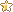Post By：2014/5/14 20:57:00

 Lo= -2318°.19281 = 201°.80719，实际上就是一个对360°求模的问题，VB里有相应语句MOD，如果不会用，可以采用笨办法，用-2318°.19281 多次加上360°，直到得到一个正数的角度，就是201°.80719。这个问题提了快两年了，怎么无人回答？5楼 个性首页 | 信息 | 搜索 | 邮箱 | 主页 | UC加好友发短信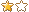Post By：2014/5/14 21:21:00

 excel:{  L0=-2318.19281=360+MOD(-2318.19281,360)= 201.80719;  }js:    {  L0=-2318.19281=360+(-2318.19281%360)= 201.80719;      }就是将负的多周角转换为正的周角.

# [求助]Vb6求儒略日算法程序和一个问题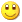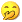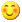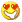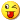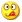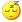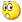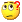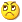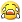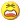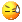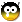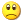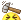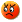签名： 不显示 显示